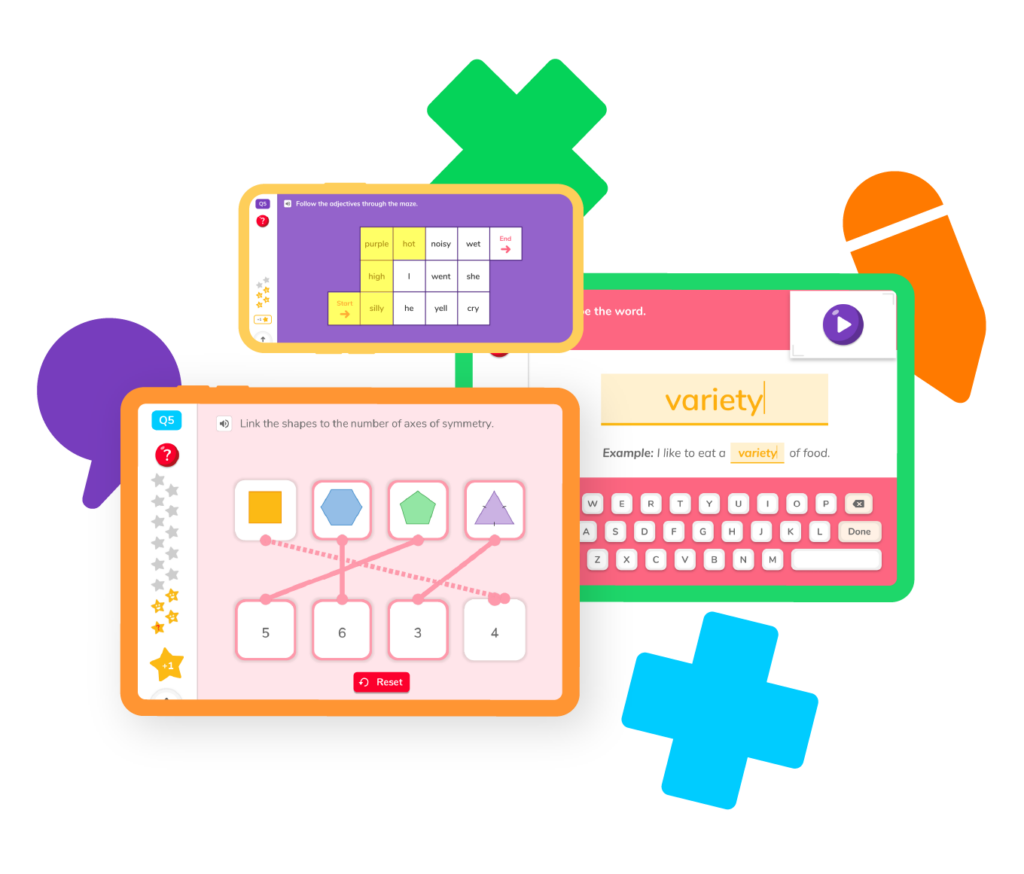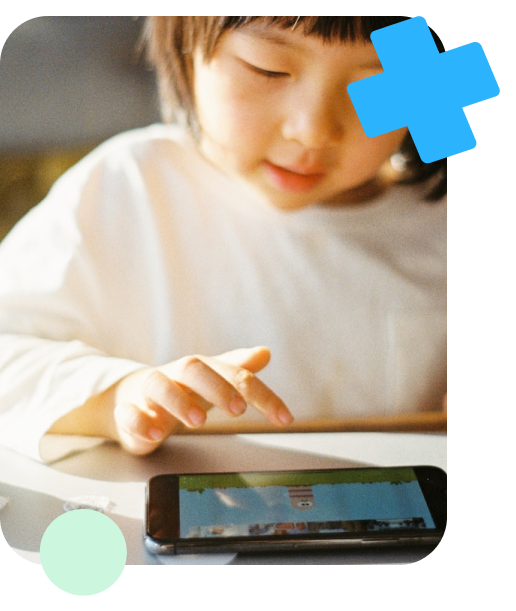# Learning Decimals

Can you guess what \$1.25 and 2.5 inches have in common? If you guessed they are both decimals, you are right! Anytime we want to represent numbers that are parts of a whole, we can use decimals. We want to learn how to use decimals well because we understand how important they are to our everyday life.## Learning How to Use DecimalsLearning to use decimals in math problems begins in the fourth grade. You will begin by learning what is a decimal and the difference between whole numbers and decimals. Then you will learn the most common place values of decimals, which will later help you to read and round them.

In fifth grade, you will extend your knowledge of decimals and will learn how to complete problems with decimals using the basic operations of adding, subtracting, multiplying, and dividing. You will complete real-life word problems with decimals and learn to convert decimals into fractions and fractions into decimals.

### When to Start?

It’s good to remember that although certain skills with decimals are emphasized in the fourth and fifth grades, all of these skills can be learned and practiced at any time. In fact, the more practice the better!

You will begin to practice addition in kindergarten. You might receive three blocks, and your teacher asks you to take two more blocks. How many blocks do you have now? You will start to practice addition problems in first grade, then get into more advanced problems in 2nd and 3rd grades.

## Decimal ResourcesLearning how to use decimals in math problems can be easy with the right tools. Join us in the Doodle Learning fun! Begin by choosing a topic about decimals. You will discover many decimal practice problems and learn some of the best decimal tips and tricks from some of the very best teachers and tutors.

## Decimal place value

Learn fun facts about decimals: how to name them, round them, and use a place value chart.

## Multiplying decimals

Practice Multiplying Decimals by whole numbers, by other decimals, and by 10, 100, and 1000.

## Dividing decimals

Explore the steps to Dividing Decimals by whole numbers and other decimals.

## Subtracting decimals

Discover how to Subtract Decimals in 4 easy steps.

## Converting decimals to fractions

Learn how to convert decimals to fractions simply by reading them.

## Converting fractions to decimals

Discover how to convert Fractions to Decimals using 2 easy methods!

## Decimal place value

Learn fun facts about decimals: how to name them, round them, and use a place value chart.

## Multiplying decimals

Practice Multiplying Decimals by whole numbers, by other decimals, and by 10, 100, and 1000.

## Dividing decimals

Explore the steps to Dividing Decimals by whole numbers and other decimals.

## Subtracting decimals

Discover how to Subtract Decimals in 4 easy steps.

## Converting decimals to fractions

Learn how to convert decimals to fractions simply by reading them.

## Converting fractions to decimals

Discover how to convert Fractions to Decimals using 2 easy methods!### What is a Decimal?

Your child may ask you, what is a decimal? Decimals are any numbers that have a decimal point. For example, the number 32.5 is a decimal because there is a decimal between 2 and 5. The decimal point separates the whole number from the parts of the number that don’t add up to a whole.

### Why are Decimals so Important?

Decimals are very important because at times an exact amount is needed. When measuring the height of a wall, or the distance traveled, even if it is not a whole number, the entire measurement needs to be represented. Decimals allow us to represent the parts that don’t make the whole and to get as close to the actual measurement as possible.

### How Do You Read a Decimal?

You can read a decimal only if you understand the place values of decimals. When reading a decimal with a whole number, you read the whole number first. Then the decimal point is read as the word “and”. Next, you read the entire number after the decimal point. Finally, whichever place value the last digit is in, will be the place value word that is said when finishing the number.

For example, 4.365 will be read as “four and three-hundred and sixty-five thousandths”.

### When Will We Use Decimals in Real-life?

The most common example of decimals in real life is our money. Any time we write our money using numbers, we use decimals. We also use decimals when we measure. If we measure the length of our garden, or the distance of a street, or the height of our siblings, we oftentimes get a number that is not completely whole. This makes learning decimals an important skill for everyone to learn.

### When Will My Child Begin Learning about Decimals?

Students begin learning about decimals in the fourth grade, where they will learn the difference between whole numbers and decimals, place values of decimals, how to read and compare decimals, and how to place decimals on a number line. It is okay to get a headstart with decimals if you feel your child is ready.

If you feel your child could use some extra decimal help, see our math app for kids in grades K-5. It was designed by teachers who understand how children learn and who have been trained in how to teach. As your child plays interactive math games and solves a variety of math problems including decimals, you will be able to track and clearly see their progress.A decimal in math is a number used to represent parts of a whole. A decimal is sometimes written by itself, like the decimal .42 or it is written attached to a whole number like the decimal 5.42.

A decimal represents the parts of a whole that don’t equal a whole number, but that are important enough to be represented.

There are many examples of decimals. The most common decimals used in math are .25, .50, .75, .33, and 3.14 the decimal that represents pi.

You read a decimal by understanding its place values. If you know the place values of a decimal, you can read it from right to left. First, you read the whole number before the decimal, if there is one. Then you say the word “and” for the decimal. Next, you read the full number after the decimal point. Followed by the place value of the last digit in.

For example, you would read the decimal 4.35 by saying, “Four and thirty-five hundredths”.

It is important to note, if there is no whole number before the decimal point, there is no need to say the word “and”. Simply read the number after the decimal point followed by the place value word.

For example, you would read the decimal .66 as “Sixty-six hundredths”.

The three most common decimal places are the tenth place, the hundredth place, and the thousandth place. If you feel your child could benefit from place value practice, see our math app for more practice problems.# Are you a parent, teacher or student?

Are you a parent or teacher?

## Hi there!

Book a chat with our team